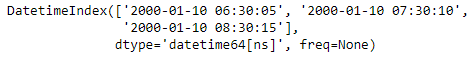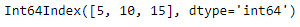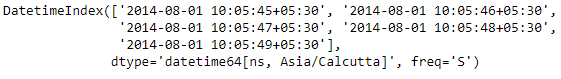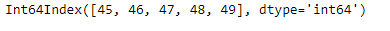Skip to content
Related Articles
Python | Pandas DatetimeIndex.second
• Difficulty Level : Expert
• Last Updated : 24 Dec, 2018

Python is a great language for doing data analysis, primarily because of the fantastic ecosystem of data-centric python packages. Pandas is one of those packages and makes importing and analyzing data much easier.

Pandas` DatetimeIndex.second` attribute outputs an Index object containing the second values present in each of the entries of the DatetimeIndex object.

Syntax: DatetimeIndex.second

Return: Index containing seconds.

Example #1: Use `DatetimeIndex.second` attribute to find the seconds value present in the DatetimeIndex object.

 `# importing pandas as pd``import` `pandas as pd`` ` `# Create the DatetimeIndex``didx ``=` `pd.DatetimeIndex([``'2000-01-10 06:30:05'``, ``'2000-01-10 07:30:10'``,``                                                ``'2000-01-10 08:30:15'``])`` ` `# Print the DatetimeIndex``print``(didx)`

Output :Now we want to find all the seconds value present in the DatetimeIndex object.

 `
``# find all the second values present in the object``didx.second`

Output :As we can see in the output, the function has returned an Index object containing the second values present in each entry of the DatetimeIndex object. Here 5, 10 and 15 are the second values in the entries of the DatetimeIndex object.

Example #2: Use `DatetimeIndex.second` attribute to find the seconds value present in the DatetimeIndex object.

 `# importing pandas as pd``import` `pandas as pd`` ` `# Create the DatetimeIndex``# Here 'S' represents secondly frequency ``didx ``=` `pd.DatetimeIndex(start ``=``'2014-08-01 10:05:45'``, freq ``=``'S'``, ``                              ``periods ``=` `5``, tz ``=``'Asia/Calcutta'``)`` ` `# Print the DatetimeIndex``print``(didx)`

Output :Now we want to find all the seconds value present in the DatetimeIndex object.

 `# find all the second values present in the object``didx.second`

Output :As we can see in the output, the function has returned an Index object containing the second values present in each entry of the DatetimeIndex object.

Attention geek! Strengthen your foundations with the Python Programming Foundation Course and learn the basics.

To begin with, your interview preparations Enhance your Data Structures concepts with the Python DS Course. And to begin with your Machine Learning Journey, join the Machine Learning – Basic Level Course

My Personal Notes arrow_drop_up